# RATE Function – Calc Int Rate – Excel, VBA, & Google Sheets

This tutorial demonstrates how to use the Excel RATE Function in Excel to calculate the interest rate of an investment.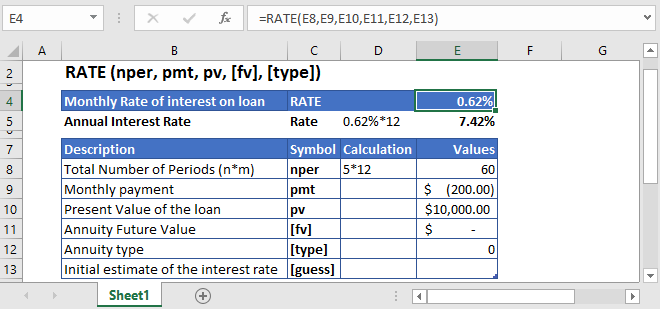## RATE Function Overview

The RATE Function Calculates the interest Rate.

To use the RATE Excel Worksheet Function, select a cell and type: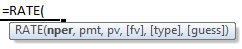(Notice how the formula inputs appear)

### RATE Function Syntax and Inputs:

nper – The total number of payment periods.

pmt – It’s the payment per period.

pv – The present value of the investment that the future payments are worth now.

fv – OPTIONAL: The Future Value of the investment or loan at the end of the number of payment periods.

type – OPTIONAL: The type argument shows when the payments are made, either at the end of the period by 0 or at the start of the period by 1. Its default value is 0.

guess – OPTIONAL: It’s an initial estimate of what the rate could be. If the [guess] argument is omitted it has a default value of 10%.

## What Is Rate?

The rate of interest period is the rate required to pay on the loan or investment. The interest rate or discounted rate is calculated only through the trial and error process.

## What Is Excel RATE Function?

RATE function is used to calculate the interest rate per period, it can either interest on a loan or rate of return on investment.

### Calculate Interest Rate of a student loan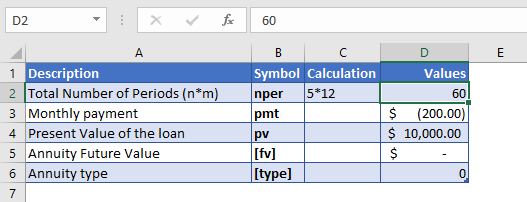Let’s find the rate of interest on a student loan of \$10,000 which has to be paid in full over the next five years. The monthly installment of the loan is \$200.

The total number of payments per period is converted into the monthly by

NPER – 5 (years) * 12 (months per year) = 60

The regular payments being made every month is with a negative sign as it represents outgoing cash

PMT = -\$200

And the future value of the investment was not mentioned in the above example, so the [fv] argument is omitted or entered zero, i.e.

FV = 0

The type of the payments isn’t mentioned also, so, the [type] argument is omitted or have default value i.e.

Type = 0

The [guess] argument is also omitted.

The formula used for the calculation of interest rate is: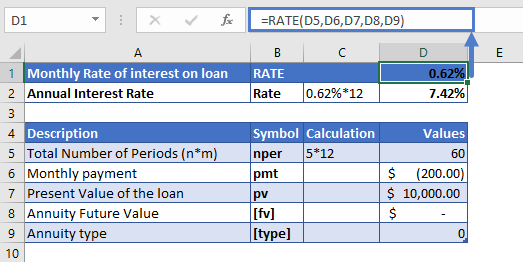The monthly interest rate calculated by using the RATE function is

RATE = 0.62%

To calculate the annual interest rate, the monthly interest rate is multiplied by 12

Annual Interest Rate = 0.62% (monthly interest rate)* 12 (total months in a year) = 7.42%

### Calculate the Interest Rate on a saving account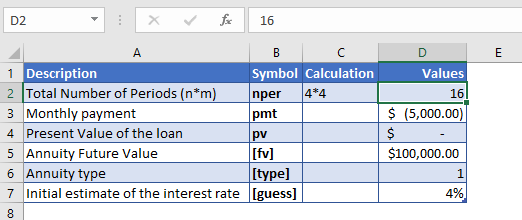Let’s calculate the annual interest rate required to save up \$100,000 in four years if the \$5,000 payments are being made at the start of every quarter with zero initial investment.

The total number of payments per period is converted into the quarterly by

NPER – 4 (years) * 4 (quarters per year) = 16

The regular payments being made every month is with a negative sign as it represents outgoing cash

PMT = -\$5,000

The present value of the investment is not mentioned in the given example

PV = 0

The formula used for the calculation is: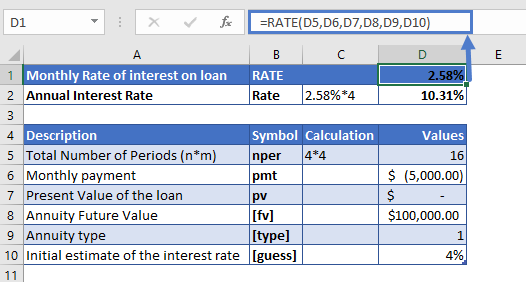The quarterly interest rate calculated by using the RATE function is

RATE = 2.58%

To calculate the annual interest rate, the quarterly interest rate is multiplied by 4

Annual Interest Rate = 2.58 % (monthly interest rate)* 4 (quarters in a year) = 10.31%

All of the above examples work exactly the same in Google Sheets as in Excel.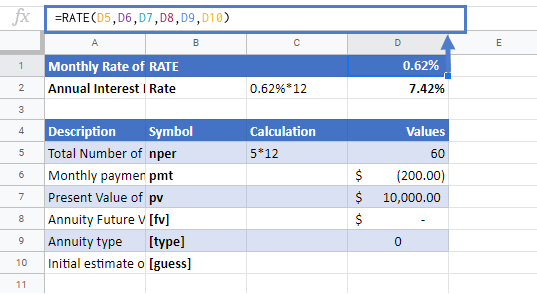Use the RATE Function to calculate the interest rate of an investment.

Make sure the units of nper and rate are consistent, i.e. in case of monthly interest rate the number of periods of investment should also be in months.

In the Financial Functions the cash outflows, such as deposits, are represented by negative numbers and the cash inflows, such as dividends, are represented by positive numbers.

The result of the RATE Function may appear as a decimal. Change the cell Number Formatting to Percentage to display the function result as a percentage:## RATE Examples in VBA

You can also use the RATE function in VBA. Type:
`application.worksheetfunction.rate(nper,pmt,pv,fv,type,guess)`
For the function arguments (nper, etc.), you can either enter them directly into the function or define variables to use instead.